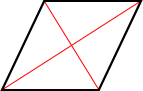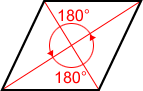# Rhombus

A rhombus is a parallelogram in which all sides are congruent. The shape of a rhombus is also often referred to as a diamond, like the suit in a standard deck of cards.

## Sides of a rhombus

All sides of a rhombus are congruent, and its opposite sides are parallel.

## Angles of a rhombus

Like a parallelogram, opposite angles of a rhombus are congruent. Also, the adjacent angles of a rhombus are supplementary, meaning that each pair of adjacent angles sums to 180°, and the sum of all the interior angles equals 360°.

## Diagonals of a rhombus

A rhombus can have two diagonals; the diagonals are perpendicular bisectors of each other.

Diagonals AC and BD are perpendicular bisectors of each other for rhombus ABCD above.

The diagonals of a rhombus bisect its angles:

Diagonals AC and BD bisect the opposite angles of rhombus ABCD above.

## Square

A square has four congruent sides and four right angles making it a special case of a rhombus. A rhombus is also a square when all its angles are right angles.

## Symmetry in a rhombus

A rhombus has 2 lines of symmetry and a rotational symmetry of order 2, which means that it can be rotated in such a way that it will look the same as the original shape 2 times in 360°.

Line of symmetryRotational symmetryBoth diagonals are lines of symmetry 180° angle of rotation about its center

## Area of a rhombus

The area of a rhombus with diagonals d1 and d2 is,Let AC = d1 and BD = d2 for rhombus ABCD above. Rhombus ABCD can be divided into triangles ABC and ADC by diagonal AC. The area of △ABC =AC×BE where BE is the altitude of △ABC. The area of △ADC =AC×DE where DE is the altitude of △ADC. The area of rhombus ABCE equals the sum of the areas of △ABC and △ADC.

 Area = Area of △ABC + △ADC =AC×BE +AC×DE =AC×(BE + DE) =AC×BD =(d1×d2)

If the length of base (b) and height (h) of a rhombus were given, the area of a rhombus is,

A = b·h